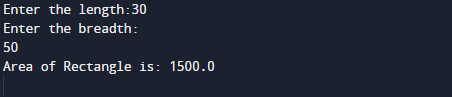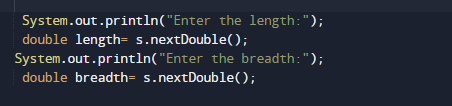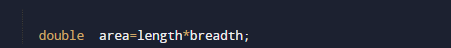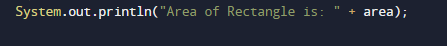# Java Program to Calculate area of rectangle

In this tutorial you will learn about the Java Program to Calculate area of rectangle and its application with practical example.

In this tutorial, we will learn to create a Java program that will Calculate Area of Rectangle using Java programming.

## Prerequisites

Before starting with this tutorial we assume that you are best aware of the following Java programming topics:

• Java Operators.
• Basic Input and Output
• Class and Object.
• Basic Java programming.

## What is area of Rectangle?

Area of any Subject(Object) is the size of a surface covered by Subject, Which you can calculate by multiplying the lengthwidth  So Area of Rectangle (area) is the product of  length ‘L’ and width or breadth ‘B’. So, Area of Rectangle = (L × B) square units.

## Java Program to Calculate area of rectangle

In this program , we will calculate Area of Rectangle of a given Length and Width .First of all user will be prompted to enter Length and Width and afterword passing these value to function to calculate Area of Rectangle.Lets have look at the program below.

## OutputIn the above program, we have first declared and initialized a set variables required in the program.

• length = it will hold entered length.
• width = it will hold entered width.
• area=  it will hold the result i.e Area or Rectangle.

Area of a Rectangle is a product of its length and breadth. So,to calculate the area of a rectangle First we will get length and breadth of the rectangle from user.As shown n image below.Now, we will calculate their product to get the area or rectangle.After, calculating their multiplication returns us the area of rectangle or given length and width,Finally we will print the area of rectangle of given values.

In this tutorial we have learn about the Java Program to Calculate area of rectangle and its application with practical example. I hope you will like this tutorial.